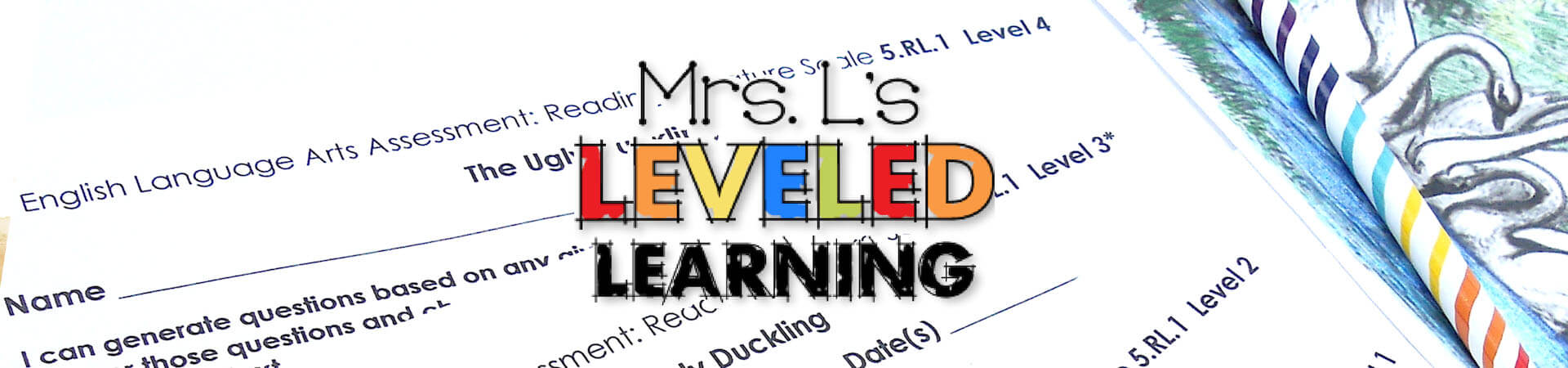Select Page

# Student Work Samples for the Math Assessments with Marzano Scales

#### After each grade level there will be links to find free sample materials. Try them out in your own classroom. It does get easier to decide what level of mastery students have after some practice. Hopefully you will find these resources make your learning journey smooth and efficient!### Grades 1-8 are available through teacherspayteachers.com. Visit the MY STORE tab above, search your grade level under “Categories.”

#### Every product page in my store has teacher comments and feedback so you can see how they’re working in other classrooms. 🙂

4.OA.3.1
In the work sample below, this student definitely shows understanding of the task and process for finding multiple possibilities, using a list. His only mistake in Scale 1 b) was that he did not recognize that repeated possibilities should not be counted in this situation. For instance, vanilla & mint, or mint & vanilla count as one possibility; order doesn’t matter. To help this student correct his mistakes, I
need to focus him on asking the question, “Do I need to count repeats?”  This standard sets kids up for noticing the difference between combinations and permutations in the middle school
standards.
In Scale 2, we have the same situation where repeats are not counted. The table is set up for students to show another accurate way to track possibilities without using a tree diagram. If a student tries using a tree diagram instead, they would end up with 4 x 3 = 12 (if they do not count double scoops of the same flavor) or 4 x 4= 16 (if they do count double scoops of the same flavor). This student accurately counted 10 possibilities using the chart to show combinations without repeats.
4.NBT.3
The Scaled Assessment for this standard is set up to show a progression of approaches to
comparing numbers. Scale 1 asks students to compare numbers to arbitrary numbers above and below itself.
Scale 2 specifically asks students to round to the nearest 10 or 100 above and below the number.
Scale 3 targets the grade level expectation that students be able to apply this idea to rounding.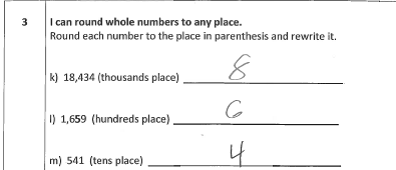In Scale 4 students are challenged to apply rounding to decimal places. This is above grade
level, and not expected in 4th grade.
This particular student had no problem mastering rounding with whole numbers, but did not make the connection to decimal places. In my next level of instruction with him I plan to lead him with questions that will help to identify the pattern in the process of rounding any place value, then ask him how he would apply the same process to decimal places. Depending on his level of conceptual understanding of decimal place values, I may have to use visual aids like Decimal Squares to help him see what tenths, hundredths, and thousandths would look like colored in. Then we can go through the same process of rounding to the nearest tenth, hundredth, etc.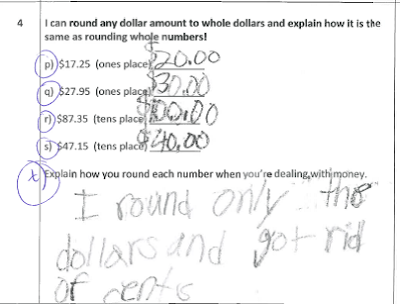4.NBT.5
In this standard, students are asked to multiply multi-digit numbers. In Scale 3 this student shows mastery of multiplication itself, but the standard also expects students to “Illustrate and explain the calculations…”.  In Scale 4 he applies the same strategies, but only accurately identifies “base ten blocks.” My next step with him is to ask him orally to explain what strategies he used and why. From there I can
decide whether to reteach him the specific strategy names, or not worry about it.
For a FREE copy of the 4th Grade Geometry Standards (4.G.1-3),
visit Mrs. L’s Leveled Learning store at the TeacherpayTeacher.com website.

For a copy of the entire Assessment including all Common Core Standards for 4th Grade Math, click HERE. You can also download the FREE PREVIEW from this page.

5.OA.2This student’s work shows that she has mastered Scales 1 & 2 of this standard; the ability to translate words into expressions.In Scale 3 and 4 however, she is making mistakes when interpreting the order of operations for each situation. In problem 3k) she reversed the x 4 and + 2, which would give an incorrect answer. 3l) is marked wrong because she incorrectly described the left and right problems that she was explaining, but I can still tell that she noticed the difference without having to calculate the problem. In Scale 4 she had more trouble describing the situation accurately. I had underlined “word problem” from the directions because she handed in the assessment with only a written expression. This was her second attempt, and from it I can see that she is still struggling to match a description to the order of operations in the expression. She uses the word “doubling” when she means x 4. For this student I decided to give her a pass on scales 1 and 2 only, until I retaught and gave her more practice opportunities for translating the order of operations correctly.

5.NBT.2

In Scales 1 and 2 for this standard, students are asked to review multiplying by groups of 10 or 100. In Scales 3 and 4, students are asked to apply their knowledge of exponents to do the same thing.This student shows mastery of the grade level standard because she passed Scale 3 with some brief but reasonable explanations. Scale 4 includes harder problems that she is not expected to master as a 5th grader, but could achieve if she noticed that she is following the same process for multiplying and dividing powers of ten. The division especially tripped her up. She lost track of how to move the decimal place in order to indicate the number of times she had divided by ten.

In the published copy of this assessment, 5th Grade Common Core Math Assessment with Marzano Scales, I revised Scale 4 so that the last three division problems would produce the same quotient, even though they have different exponents of ten. I also revised the question so that children would have to notice and explain why they got the same quotient each time.

I created a game for the SMARTboard in a .notebook file to help students understand the movement of the decimal place when multiplying and dividing numbers by exponents of ten. Actually, the game asks students to move the digits of their number on a place value chart, so they make the connection that it is really the digits that move to different places, not the decimal that moves. In class I point out these connections and check to see if they can start applying them to larger numbers, such as in Scale 4. The game is called Place Value Volley, and it has a few different levels using different notation for multiples of ten, exponents, and metric system conversions, because they work the same way.

5.NBT.1

Sometimes a student doesn’t ‘pass’ every scale, but still gets an A from me. In the example below, the work in Scale 1 is incomplete because the ten and hundred are not accurately represented. I made a note of this to the student, but since he had no problem completing Scales 2-4 accurately, I know he mastered this standard and we moved on.

For a FREE copy of the 5th Grade Operations and Algebraic Thinking Standards (5.OA.1-3),
visit Mrs. L’s Leveled Learning store at the TeacherpayTeacher.com website.

For a copy of the entire Assessment including all Common Core Standards for 5th Grade Math, click HERE. You can also download the FREE PREVIEW from this page.
*Note: The revised and published version of the 5th Grade Math Assessments with Marzano Scales include an answer column to the left of each work space, for a quick check of multiple students at one glance. An answer key is also provided at the end of the document.

6.RP.3
Subskills a. and b. build on 5th grade skills (see 5.OA.3) of plotting x and y coordinates using a table of values based on ratios.  In Scale 2 I ask students to “have a ‘y’ value for every ‘x’ value on the grid.” his student overlooked this direction and only plotted the x and y values from the table above.  Yes, he plotted the points correctly, so I could confidently say that he had passed scale 2, but in this situation I will ask him to add the missing values so I can see him applying the correct ratio to new x values.
Subskills c. and d. are addressed in Scales 3 and 4 of this assessment. In problem 3d) the student correctly calculated an additional time of 176 miles. You might notice that the published version has been revised so students solve for 176 miles total, instead of 165 + 176 miles. Problem 3e) was miscalculated because his work shows that he was trying to find out how many times 15 went into 100%, but it doesn’t work out evenly, so his strategy got him close, but not to the accurate answer of \$25. My strategy with this student is to review strategies for checking his work with percents; working backwards, or using equivalent fractions to check that the part to whole ratio of the percents match his solution. For instance, if he had compared 15/100 to \$3.75/\$24, he would see that \$3.75 x 4 makes 15, but 24 x 4 does not make 100! Since this is the only problem that was incorrect in Scale 3, and he gave a decent answer for Scale 4, I am confident that he has mastered this standard, but still needs some follow up as far as checking his work.

##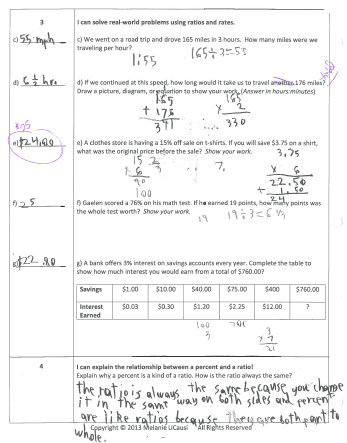6.NS.1
To create the Scale 1 and 2 for this standard, I cited 4.NBT.6 and 5.NF.7 as previous standards that students can connect to dividing fractions. The first idea is that multiplication and division are inverse operations that can be used to check each other. This is helpful when students are just starting to understand division and fractions and may be tempted to guess answers because they can’t visualize what’s going on. Scale 2 reminds students to reflect on the meaning of multiplication and division of fractions by whole numbers. The directions specifically ask students to “draw a picture” of each problem because the standard also emphasizes using visual models. This student tried to convert the fractions to decimals instead, and wasn’t able to see that she was making mistakes. If she had drawn a picture of 1/4 of a whole, then divided that into 5 equal pieces, she would have easily recognized that 20 pieces would fit into 1 whole = 1/20.

When I moved from teaching 1st grade, to 3-4-5-6 math classes, I had to spend a little time understanding division of fractions myself. Drawing models helped me to understand how a fraction could be broken into another fraction. The visual aspect here is essential! Especially because Scale 3, which is the target for this standard, asks students to represent division of fractions by fractions, which is even more complicated than whole numbers. Here she tried to simply divide by 2, and then 5, which could have worked if she had accurately divided 16 oz. into 10 equal pieces, and created a simplified fraction. For instance, if 16oz. divided by 10 is equal to 1.6 oz., then 1.6/16 = 1/10.

6.NS.4
Here students are asked to use skills they have from 5th grade (multiples, factors, and distributive property) in specific ways. This student undoubtedly shows mastery of this standard. I chose this one as an example of a good explanation for question 3p).
6.NS.6
The answer key included in the published versions of the assessment do not have many pictures, so here’s a quick visual of an accurate grid. It helps me to grade faster if I don’t have to reread the coordinates for every point. You’ll notice that Scale 4 is not included for this standard because there is so much to fit into Scale 3. I thought the workspace was more important than inventing an extension.6.NS.7
In 6.NS.6, students are asked to apply an understanding of negative integers to coordinate grids. In 6.NS.7, they must apply it to new contexts such as story problems. Students need to be able to identify the context for positive, negative, and absolute values, but aren’t required to solve operations yet. That is why Scale 4, shows tasks above grade level: computation with integers. This student shows mastery of the standard, and the ability to apply this understanding to adding and subtracting integers. The diagrams of sea level help them to visualize the position of the integers, like a number line would. An extension to these tasks might be asking students to draw their own diagram based on a story problem, then check to see if they interpreted the position of the numbers correctly.
For a FREE copy of the 6th Grade Ratios and Proportional Relationships Standards (6.RP.1-3),
visit Mrs. L’s Leveled Learning store at the TeacherpayTeacher.com website.

For a copy of the entire Assessment including all Common Core Standards for 6th Grade Math, click HERE. You can also download the FREE PREVIEW from this page.
*Note: The revised and published version of the 6th Grade Math Assessment with Marzano Scales include an answer column to the left of each work space, for a quick check of multiple students at one glance. An answer key is also provided at the end of the document.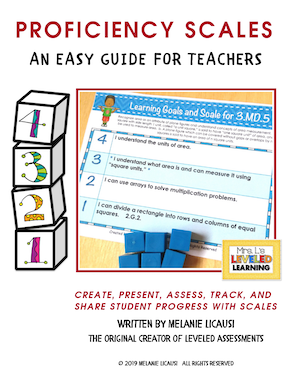## Subscribe NOW!

Get immediate access to my GUIDE to EASY Proficiency Scales from a teacher's point of view!